Home | | Maths 9th std | Exercise 1.5: De MorganŌĆÖs Laws

# Exercise 1.5: De MorganŌĆÖs Laws

Maths : Set Language : Book Back, Exercise, Example Numerical Question with Answers, Solution: Exercise 1.5: De MorganŌĆÖs Laws

Exercise 1.5

1. Using the adjacent Venn diagram, find the following sets:(i) A ŌłÆ B (ii) B -C (iii) AŌĆ▓ Ōł¬ BŌĆ▓  (iv) AŌĆ▓ Ōł® BŌĆ▓ (v) (B Ōł¬C)ŌĆ▓  (vi) A ŌłÆ (B Ōł¬C) (vii) A ŌłÆ (B Ōł®C)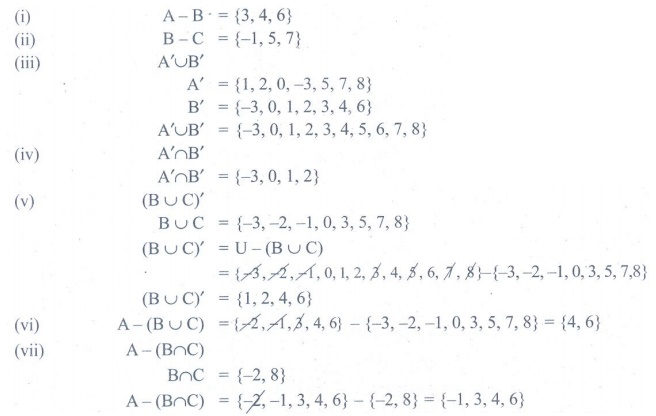2. If K = { a , b, d, e, f } , L = { b, c, d, g } and M = { a , b, c, d, h } , then find the following:

(i) K Ōł¬ (L Ōł® M) (ii) K Ōł® (L Ōł¬ M) (iii) (K Ōł¬ L ) Ōł® (K Ōł¬ M) (iv) (KŌł® L) Ōł¬ (K Ōł® M)

and verify distributive laws.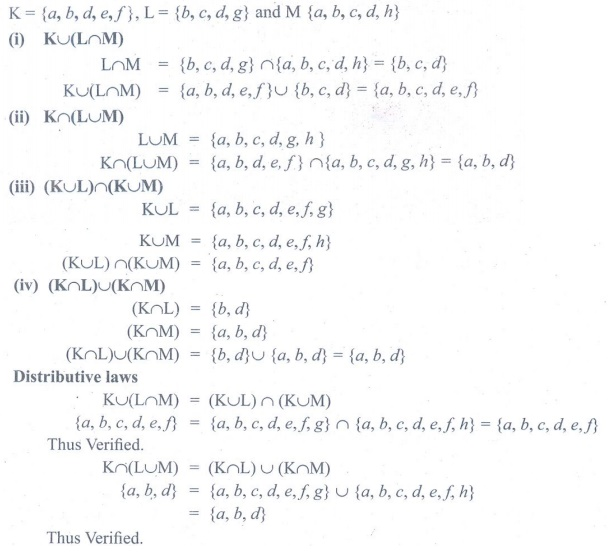3. If A = {x : x Ōłł Ōäż, ŌłÆ 2 < x Ōēż 4}, B={x : x Ōłł W , x Ōēż 5}, C = {ŌłÆ4, ŌłÆ1, 0, 2, 3, 4}, then  verify A Ōł¬ (B Ōł®C ) = (A Ōł¬ B ) Ōł® (A Ōł¬C) .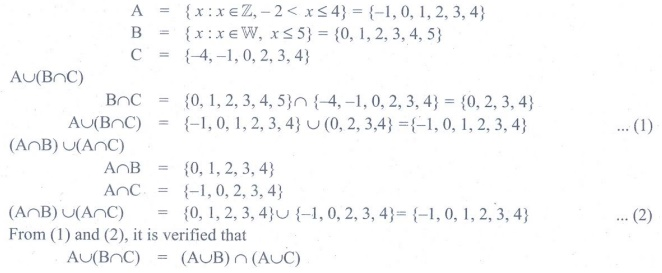4. Verify A Ōł¬ (B Ōł®C ) = (A Ōł¬ B ) Ōł® (A Ōł¬C) using Venn diagrams.5. If A = {b, c, e, g, h} , B = {a , c, d , g, i } and C = {a , d, e, g, h} , then show that AŌłÆ(B Ōł®C) = (AŌłÆB)Ōł¬(AŌłÆC).6. If A = {x : x = 6n, nŌłłW and n<6}, B = {x : x = 2n, n Ōłł ŌäĢ and 2<nŌēż9} and C = {x : x = 3n, n Ōłł ŌäĢ and 4Ōēżn<10}, then show that A ŌłÆ (B Ōł® C ) = (A ŌłÆ B ) Ōł¬ (A ŌłÆC)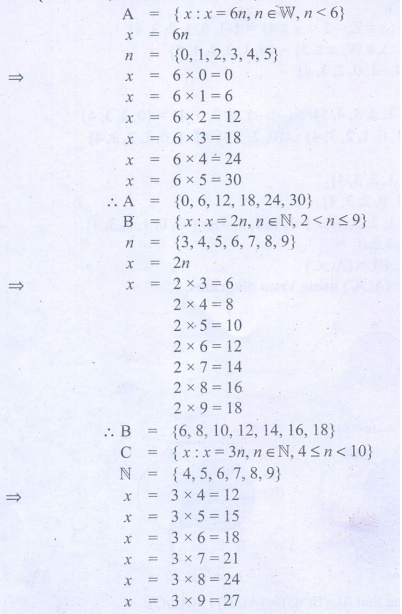7. If A = {ŌĆō2,0,1,3,5}, B = {ŌĆō1,0,2,5,6} and C = {ŌĆō1,2,5,6,7}, then show that A ŌłÆ (B Ōł¬C) = (AŌłÆB)Ōł®(AŌłÆC).8. If A = {y : y = [a + 1] /2 , aŌłłW and a Ōēż 5}, B = {y : y = [2n -1] / 2, nŌłłW and n < 5} and  C = {-1, -1/2,1,3/2,2}, then show that A ŌłÆ (B Ōł¬ C ) = (A ŌłÆ B ) Ōł® (A ŌłÆC) .9. Verify A ŌłÆ (B Ōł® C ) = (A ŌłÆ B ) Ōł¬ (A ŌłÆC) using Venn diagrams.10. If U = {4,7,8,10,11,12,15,16}, A={7,8,11,12} and B = {4,8,12,15} then verify De MorganŌĆÖs Laws for complementation.11. Verify (A Ōł® B)ŌĆ▓ = AŌĆ▓ Ōł¬ BŌĆ▓ using Venn diagrams.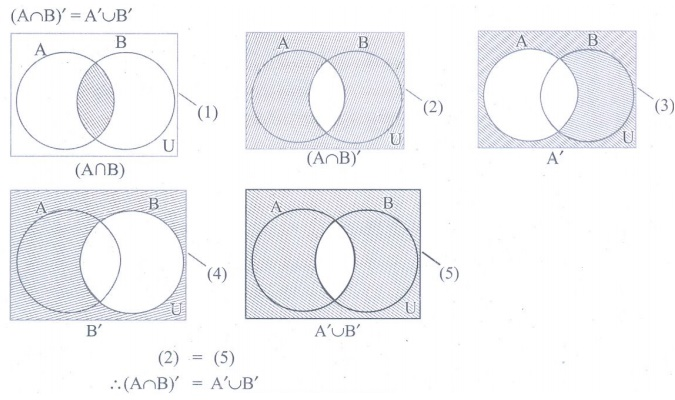Tags : Numerical Problems with Answers, Solution | Set Language | Maths , 9th Maths : UNIT 1 : Set Language
Study Material, Lecturing Notes, Assignment, Reference, Wiki description explanation, brief detail
9th Maths : UNIT 1 : Set Language : Exercise 1.5: De MorganŌĆÖs Laws | Numerical Problems with Answers, Solution | Set Language | Maths